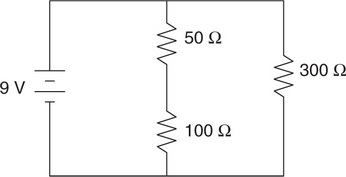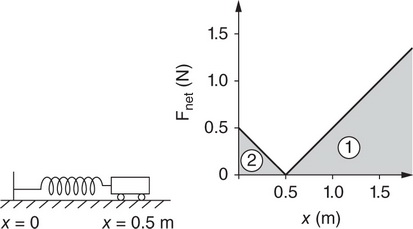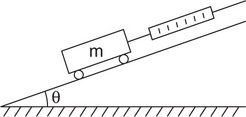# AP Physics 1 Practice Test 16

### Test Information10 questions18 minutes

1.A person pulls on a string, causing a block to move to the left at a constant speed. The free body diagram shows the four forces acting on the block: the tension (T) in the string, the normal force (Fn), the weight (W), and the friction force (Ff). The coefficient of friction between the block and the table is 0.30.

Which is the Newton’s third law force pair to T?

2.A person pulls on a string, causing a block to move to the left at a constant speed. The free body diagram shows the four forces acting on the block: the tension (T) in the string, the normal force (Fn), the weight (W), and the friction force (Ff). The coefficient of friction between the block and the table is 0.30.

Which of the following correctly ranks the four forces shown?

3. Which of the following circuits shows a placement of meters and an observation of their readings that would allow researchers to experimentally demonstrate whether energy is conserved in the circuit?

4.Question below refers to the circuit shown in the figure, which includes a 9 V battery and three resistors.

Which of the following ranks the resistors by the charge that flows through each in a given time interval?

5.Question below refers to the circuit shown in the figure, which includes a 9 V battery and three resistors.

What is the voltage across the 50 Ω resistor?

6. The charge on an oil drop is measured in the laboratory. Which of the following measurements should be rejected as highly unlikely to be correct?

7.A cart attached to a spring initially moves in the x direction at a speed of 0.40 m/s. The spring is neither stretched nor compressed at the cart’s initial position (x = 0.5 m). The figure shows a graph of the magnitude of the net force experienced by the cart as a function of x, with two areas under the graph labeled. Is it possible to analyze the graph to determine the change in the cart’s kinetic energy as it moves from its initial position to x = 1.5 m?

8. A horse is attached to a cart that is at rest behind it. Which force, or combination of forces, explains how the horse-cart system can accelerate from rest?

9. A pipe full of air is closed at one end. A standing wave is produced in the pipe, causing the pipe to sound a note. Which of the following is a correct statement about the wave’s properties at the closed end of the pipe?

10.In the laboratory, a cart of mass m is held in place on a smooth incline by a rope attached to a spring scale, as shown in the figure. The angle of the incline from the horizontal θ varies between 0° and 90°. A graph of the reading in the spring scale as a function of the angle θ is produced. Which of the following will this graph look like?